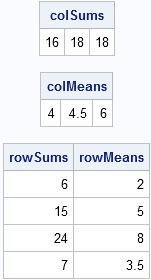Writing efficient SAS/IML programs is very important. One aspect to efficient SAS/IML programming is to avoid unnecessary DO loops. In my book, Statistical Programming with SAS/IML Software, I wrote (p. 80):

One way to avoid writing unnecessary loops is to take full advantage of the subscript reduction operators for matrices. These operators enable you to perform common statistical operations (such as sums, means, and sums of squares) on either the rows or the columns of a matrix.

For example, the following statements compute the sum and mean of columns and of rows for a matrix:

```proc iml; /** compute sum and mean of each column **/ x = {1 2 3, 4 5 6, 7 8 9, 4 3 .}; colSums = x[+, ]; colMeans = x[:, ]; rowSums = x[ ,+]; rowMeans = x[ ,:]; print colSums, colMeans, rowSums rowMeans;```As I explain in my book, the expression x[+, ] uses the "+" subscript operator to "reduce" the matrix by summing the elements of each row for all columns. (Recall that not specifying a column in the second subscript is equivalent to specifying all columns.) The expression x[:, ] uses the ":" subscript operator to compute the mean for each column. The row sums and means are computed similarly. Notice that the subscript reduction operators correctly handle the missing value in the third column.

A common use of subscript reduction operators is to compute the marginal frequencies in a two-way frequency table. This is the origin of the name "reduction operator."

See the SAS/IML User's Guide for more details on subscript reduction operators, including a complete list of operators. You might also want to learn how to use the results of subscript reduction operations in computations.

Share